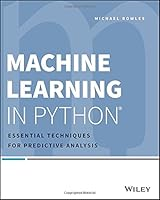# Machine Learning in Python: Essential Techniques for Predictive Analysis0 Reviews
2015-04-20
360 pages

## Book Description

Learn a simpler and more effective way to analyze data and predict outcomes with Python

in Python shows you how to successfully analyze data using only two core algorithms, and how to apply them using Python. By focusing on two algorithm families that effectively predict outcomes, this book is able to provide full descriptions of the mechanisms at work, and the examples that illustrate the machinery with specific, hackable . The algorithms are explained in simple terms with no complex math and applied using Python, with guidance on algorithm selection, data preparation, and using the trained models in practice. You will learn a core set of Python techniques, various methods of building predictive models, and how to measure the performance of each model to ensure that the right one is used. The chapters on penalized linear regression and ensemble methods dive deep into each of the algorithms, and you can use the sample code in the book to develop your own data analysis solutions.

Machine learning algorithms are at the core of data and visualization. In the past, these methods required a deep background in math and statistics, often in combination with the specialized R . This book demonstrates how machine learning can be implemented using the more widely used and accessible Python . * Predict outcomes using linear and ensemble algorithm families * Build predictive models that solve a range of simple and complex problems * Apply core machine learning algorithms using Python * Use sample code directly to build custom solutions

Machine learning doesn't have to be complex and highly specialized. Python makes this more accessible to a much wider audience, using methods that are simpler, effective, and well tested. Machine Learning in Python shows you how to do this, without requiring an extensive background in math or statistics.

Chapter 1 The Two Essential Algorithms for Making Predictions
Chapter 2 Understand the Problem by Understanding the Data
Chapter 3 Predictive Model Building: Balancing Performance, Complexity, and Big Data
Chapter 4 Penalized Linear Regression
Chapter 5 Building Predictive Models Using Penalized Linear Methods
Chapter 6 Ensemble Methods
Chapter 7 Building Ensemble Models with Python

## Book Details

• Title: Machine Learning in Python: Essential Techniques for Predictive Analysis
• Author:
• Length: 360 pages
• Edition: 1
• Language: English
• Publisher:
• Publication Date: 2015-04-20
• ISBN-10: 1118961749
• ISBN-13: 9781118961742